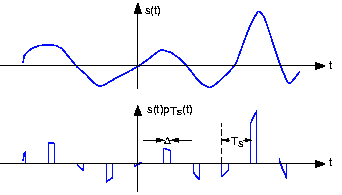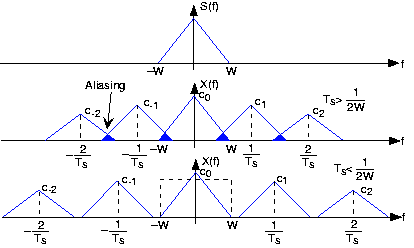# 5.3: The Sampling Theorem

$$\newcommand{\vecs}{\overset { \rightharpoonup} {\mathbf{#1}} }$$ $$\newcommand{\vecd}{\overset{-\!-\!\rightharpoonup}{\vphantom{a}\smash {#1}}}$$$$\newcommand{\id}{\mathrm{id}}$$ $$\newcommand{\Span}{\mathrm{span}}$$ $$\newcommand{\kernel}{\mathrm{null}\,}$$ $$\newcommand{\range}{\mathrm{range}\,}$$ $$\newcommand{\RealPart}{\mathrm{Re}}$$ $$\newcommand{\ImaginaryPart}{\mathrm{Im}}$$ $$\newcommand{\Argument}{\mathrm{Arg}}$$ $$\newcommand{\norm}{\| #1 \|}$$ $$\newcommand{\inner}{\langle #1, #2 \rangle}$$ $$\newcommand{\Span}{\mathrm{span}}$$ $$\newcommand{\id}{\mathrm{id}}$$ $$\newcommand{\Span}{\mathrm{span}}$$ $$\newcommand{\kernel}{\mathrm{null}\,}$$ $$\newcommand{\range}{\mathrm{range}\,}$$ $$\newcommand{\RealPart}{\mathrm{Re}}$$ $$\newcommand{\ImaginaryPart}{\mathrm{Im}}$$ $$\newcommand{\Argument}{\mathrm{Arg}}$$ $$\newcommand{\norm}{\| #1 \|}$$ $$\newcommand{\inner}{\langle #1, #2 \rangle}$$ $$\newcommand{\Span}{\mathrm{span}}$$

##### Learning Objectives
• Converting between a signal and numbers.

## Analog-to-Digital Conversion

Because of the way computers are organized, signal must be represented by a finite number of bytes. This restriction means that both the time axis and the amplitude axis must be quantized: They must each be a multiple of the integers. 1 Quite surprisingly, the Sampling Theorem allows us to quantize the time axis without error for some signals. The signals that can be sampled without introducing error are interesting, and as described in the next section, we can make a signal "samplable" by filtering. In contrast, no one has found a way of performing the amplitude quantization step without introducing an unrecoverable error. Thus, a signal's value can no longer be any real number. Signals processed by digital computers must be discrete-valued: their values must be proportional to the integers. Consequently, analog-to-digital conversion introduces error.

## The Sampling Theorem

Digital transmission of information and digital signal processing all require signals to first be "acquired" by a computer. One of the most amazing and useful results in electrical engineering is that signals can be converted from a function of time into a sequence of numbers without error: We can convert the numbers back into the signal with (theoretically) no error. Harold Nyquist, a Bell Laboratories engineer, first derived this result, known as the Sampling Theorem, in the 1920s. It found no real application back then. Claude Shannon, also at Bell Laboratories, revived the result once computers were made public after World War II.

The sampled version of the analog signal s(t) is s(nTs), with Ts known as the sampling interval. Clearly, the value of the original signal at the sampling times is preserved; the issue is how the signal values between the samples can be reconstructed since they are lost in the sampling process. To characterize sampling, we approximate it as the product:

$x(t)=s(t)P_{T_{s}}(t) \nonumber$

with PTs being the periodic pulse signal. The resulting signal, as shown in Figure 5.3.1 has nonzero values only during the time intervals :

$\left ( nT_{s}-\frac{\Delta }{2}, nT_{s}+\frac{\Delta }{2}\right ),n\in \left \{ ...,-1,0,1,... \right \} \nonumber$Figure 5.3.1 The waveform of an example signal is shown in the top plot and its sampled version in the bottom.

For our purposes here, we center the periodic pulse signal about the origin so that its Fourier series coefficients are real (the signal is even).

$P_{T_{s}}(t)=\sum_{k=-\infty }^{\infty }c_{k}e^{\frac{i2\pi kt}{T_{s}}} \nonumber$

$c_{k}=\frac{\sin \left ( \frac{\pi k\Delta }{T_{s}}\right )}{\pi k} \nonumber$

If the properties of s(t) and the periodic pulse signal are chosen properly, we can recover s(t) from x(t) by filtering.

To understand how signal values between the samples can be "filled" in, we need to calculate the sampled signal's spectrum. Using the Fourier series representation of the periodic sampling signal,

$x(t)=\sum_{k=-\infty }^{\infty }c_{k}e^{\frac{i2\pi kt}{T_{s}}}s(t) \nonumber$

Considering each term in the sum separately, we need to know the spectrum of the product of the complex exponential and the signal. Evaluating this transform directly is quite easy.

$\int_{-\infty }^{\infty }s(t)e^{\frac{i2\pi kt}{T_{s}}}e^{i2\pi ft}dt=\int_{-\infty }^{\infty }s(t)e^{-\left ( i2\pi \left ( f-\frac{k}{T_{s}} \right )\right )}dt=S\left ( f-\frac{k}{T_{s}} \right ) \nonumber$

Thus, the spectrum of the sampled signal consists of weighted (by the coefficients ck) and delayed versions of the signal's spectrum Figure 5.3.2 below.

$X(f)=\sum_{-\infty }^{\infty }c_{k}S\left ( f-\frac{k}{T_{s}} \right ) \nonumber$

In general, the terms in this sum overlap each other in the frequency domain, rendering recovery of the original signal impossible. This unpleasant phenomenon is known as aliasing.Figure 5.3.2 The spectrum of some bandlimited (to W Hz) signal is shown in the top plot. If the sampling interval Ts is chosen too large relative to the bandwidth W, aliasing will occur. In the bottom plot, the sampling interval is chosen sufficiently small to avoid aliasing. Note that if the signal were not bandlimited, the component spectra would always overlap.

If, however, we satisfy two conditions:

• The signal s(t) is bandlimited—has power in a restricted frequency range—to W Hz
• the sampling interval Ts is small enough so that the individual components in the sum do not overlap - Ts < 1/2 W

Aliasing will not occur. In this delightful case, we can recover the original signal by lowpass filtering x(t) with a filter having a cutoff frequency equal to W Hz. These two conditions ensure the ability to recover a bandlimited signal from its sampled version: We thus have the Sampling Theorem.

##### Exercise $$\PageIndex{1}$$

The Sampling Theorem (as stated) does not mention the pulse width Δ. What is the effect of this parameter on our ability to recover a signal from its samples (assuming the Sampling Theorem's two conditions are met)?

Solution

The only effect of pulse duration is to unequally weight the spectral repetitions. Because we are only concerned with the repetition centered about the origin, the pulse duration has no significant effect on recovering a signal from its samples.

The frequency 1/2Ts, known today as the Nyquist frequency and the Shannon sampling frequency, corresponds to the highest frequency at which a signal can contain energy and remain compatible with the Sampling Theorem. High-quality sampling systems ensure that no aliasing occurs by unceremoniously lowpass filtering the signal (cutoff frequency being slightly lower than the Nyquist frequency) before sampling. Such systems therefore vary the anti-aliasing filter's cutoff frequency as the sampling rate varies. Because such quality features cost money, many sound cards do not have anti-aliasing filters or, for that matter, post-sampling filters. They sample at high frequencies, 44.1 kHz for example, and hope the signal contains no frequencies above the Nyquist frequency (22.05 kHz in our example). If, however, the signal contains frequencies beyond the sound card's Nyquist frequency, the resulting aliasing can be impossible to remove.

##### Exercise $$\PageIndex{1}$$

To gain a better appreciation of aliasing, sketch the spectrum of a sampled square wave. For simplicity consider only the spectral repetitions centered at $-\frac{1}{T_{s}},0,\frac{1}{T_{s}} \nonumber$
Let the sampling interval Ts be 1; consider two values for the square wave's period: 3.5 and 4. Note in particular where the spectral lines go as the period decreases; some will move to the left and some to the right. What property characterizes the ones going the same direction?

Solution

The square wave's spectrum is shown by the bolder set of lines centered about the origin. The dashed lines correspond to the frequencies about which the spectral repetitions (due to sampling with Ts = 1 ) occur. As the square wave's period decreases, the negative frequency lines move to the left and the positive frequency ones to the right.

If we satisfy the Sampling Theorem's conditions, the signal will change only slightly during each pulse. As we narrow the pulse, making

##### Exercise $$\PageIndex{1}$$

What is the simplest bandlimited signal? Using this signal, convince yourself that less than two samples/period will not suffice to specify it. If the sampling rate 1/Ts is not high enough, what signal would your resulting undersampled signal become?

Solution

The simplest bandlimited signal is the sine wave. At the Nyquist frequency, exactly two samples/period would occur. Reducing the sampling rate would result in fewer samples/period, and these samples would appear to have arisen from a lower frequency sinusoid.

##### Footnotes
1. We assume that we do not use floating-point A/D converters.

This page titled 5.3: The Sampling Theorem is shared under a CC BY 1.0 license and was authored, remixed, and/or curated by Don H. Johnson via source content that was edited to the style and standards of the LibreTexts platform; a detailed edit history is available upon request.